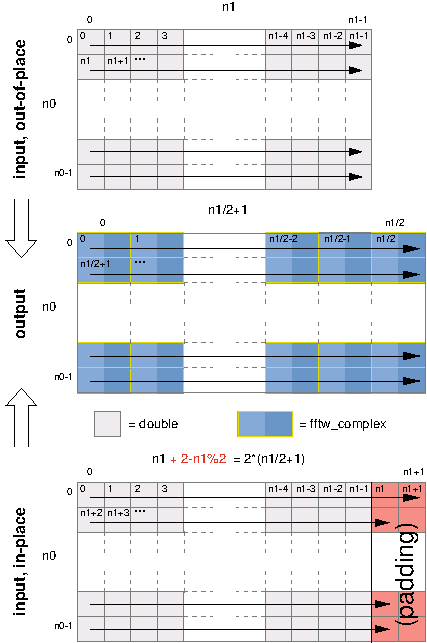Next: , Previous: , Up: Tutorial   [Contents][Index]

### 2.4 Multi-Dimensional DFTs of Real Data

Multi-dimensional DFTs of real data use the following planner routines:

```fftw_plan fftw_plan_dft_r2c_2d(int n0, int n1,
double *in, fftw_complex *out,
unsigned flags);
fftw_plan fftw_plan_dft_r2c_3d(int n0, int n1, int n2,
double *in, fftw_complex *out,
unsigned flags);
fftw_plan fftw_plan_dft_r2c(int rank, const int *n,
double *in, fftw_complex *out,
unsigned flags);
```

as well as the corresponding `c2r` routines with the input/output types swapped. These routines work similarly to their complex analogues, except for the fact that here the complex output array is cut roughly in half and the real array requires padding for in-place transforms (as in 1d, above).

As before, `n` is the logical size of the array, and the consequences of this on the the format of the complex arrays deserve careful attention. Suppose that the real data has dimensions n0 × n1 × n2 × … × nd-1 (in row-major order). Then, after an r2c transform, the output is an n0 × n1 × n2 × … × (nd-1/2 + 1) array of `fftw_complex` values in row-major order, corresponding to slightly over half of the output of the corresponding complex DFT. (The division is rounded down.) The ordering of the data is otherwise exactly the same as in the complex-DFT case.

For out-of-place transforms, this is the end of the story: the real data is stored as a row-major array of size n0 × n1 × n2 × … × nd-1 and the complex data is stored as a row-major array of size n0 × n1 × n2 × … × (nd-1/2 + 1) .

For in-place transforms, however, extra padding of the real-data array is necessary because the complex array is larger than the real array, and the two arrays share the same memory locations. Thus, for in-place transforms, the final dimension of the real-data array must be padded with extra values to accommodate the size of the complex data—two values if the last dimension is even and one if it is odd. That is, the last dimension of the real data must physically contain 2 * (nd-1/2+1) `double` values (exactly enough to hold the complex data). This physical array size does not, however, change the logical array size—only nd-1 values are actually stored in the last dimension, and nd-1 is the last dimension passed to the plan-creation routine.

For example, consider the transform of a two-dimensional real array of size `n0` by `n1`. The output of the r2c transform is a two-dimensional complex array of size `n0` by `n1/2+1`, where the `y` dimension has been cut nearly in half because of redundancies in the output. Because `fftw_complex` is twice the size of `double`, the output array is slightly bigger than the input array. Thus, if we want to compute the transform in place, we must pad the input array so that it is of size `n0` by `2*(n1/2+1)`. If `n1` is even, then there are two padding elements at the end of each row (which need not be initialized, as they are only used for output).

The following illustration depicts the input and output arrays just described, for both the out-of-place and in-place transforms (with the arrows indicating consecutive memory locations):These transforms are unnormalized, so an r2c followed by a c2r transform (or vice versa) will result in the original data scaled by the number of real data elements—that is, the product of the (logical) dimensions of the real data.

(Because the last dimension is treated specially, if it is equal to `1` the transform is not equivalent to a lower-dimensional r2c/c2r transform. In that case, the last complex dimension also has size `1` (`=1/2+1`), and no advantage is gained over the complex transforms.)

Next: , Previous: , Up: Tutorial   [Contents][Index]